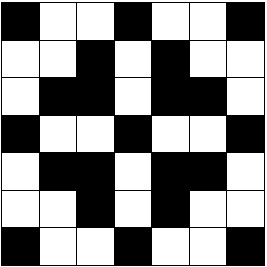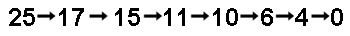## 2006 Qualifying Quiz

You may also be interested in the printable (PDF) format.

1. In the following 7 × 7 grid, each square is colored black or white. This particular coloring has a lot of symmetries: the pattern of black and white squares is unchanged if the grid is rotated by 90°, 180°, or 270°, or if it is reflected in a horizontal, vertical, or diagonal line through the center. How many ways are there to color a 7 × 7 grid black and white with these same symmetries? How about an N × N grid?2. (Contributed by Khoa Lu Nguyen, Mathcamp 2005) Is it possible to find 2006 distinct points in the plane so that for any two of the points P and Q, the circle with diameter PQ goes through at least one more point from the set?

3. Two sets A and B have the property that there are precisely 144 sets which are subsets of either A or B or both. How many elements are in the union AB? (Hint: The set of subsets of a set S is called the power set of S. You may want to look it up if you've never encountered it before. Of course, if you do, you should reference your source in your solution.)

4. Arda and Cordelia are playing a game. Arda writes down a positive integer. Cordelia looks at it and decides whether she wants to go first or second. The players then take turns subtracting powers of 2 (i.e. 1, 2, 4, 8, 16, ...) from Arda's number. Negative numbers are not allowed, and the player who gets to 0 wins. For instance, a typical game might go as follows:1. For which numbers written by Arda should Cordelia choose to go first, and what should her strategy be?
2. What if the players must subtract powers of 3 instead of powers of 2?
3. What if they subtract powers of a fixed positive integer k?

5. Start with four numbers arranged in a circle. Between each pair of adjacent numbers, write their average. Now erase the original numbers, so that once again you are left with four numbers in a circle. You can repeat this process as many times as you wish. (For example, if the original numbers were 1, 3, 7, 5 in that order, then after one iteration you get 2, 5, 6, 3; after two iterations, you get 7/2, 11/2, 9/2, 5/2, etc.)

Suppose after 20 iterations, you obtain the numbers 1, 2, 3, 4, not necessarily in that order. Can you tell what numbers you had after one iteration? Can you tell what the original numbers were?

6. What is the maximum number of 90° angles that an n-sided polygon can have? The polygon need not be convex, but you have to distinguish between 90° and 270° angles. For instance, a hexagon in the shape of the letter L has five 90° angles and one 270° angle.

7. Order the numbers 1, 2, ... , n any way you want. Add up the differences between successive numbers, with all differences counted positively: for example, if you start with the ordering 5, 3, 1, 4, 2, you get 2 + 2 + 3 + 2 = 9. What is the largest number you can obtain in this way? Your answer, of course, will depend on n. (Be sure to prove that the number you get really is the largest possible!)

8. The Rainbow Game is played by a team of seven. Each player gets a hat, which can be any one of the seven colors red, orange, yellow, green, blue, indigo, and violet. The colors of the hats are independent of each other and repetitions are allowed: for instance, it may happen that all the hats are green. Each player can see only the colors of the six hats worn by the rest of the team; no player can see the color of his or her own hat. The players are to guess the colors of their own hats, and if at least one player guesses correctly then the team as a whole wins.

The players may not communicate in any way during the game, and they must all announce their guesses simultaneously. They are, however, allowed to plan out a strategy in advance, and they hope to find a strategy which will guarantee them success for every possible arrangement of hats. Is there such a strategy? Either find one or show that it cannot exist. (As a warm-up, try two players and two colors, or three players and three colors.)

9. Let's define a curious sort of "distance" on the positive integers. We say that two positive integers a and b are one step apart if ab+1 is a perfect square; for instance, 2 and 24 are one step apart. If we can find a sequence of positive integers

a = c0, c1,..., cn = b,

such that ci and ci+1 are one step apart for each i, we say that it is possible to go from a to b in n steps. If n is the smallest positive integer such that it is possible to go from a to b in n steps, then we say that a and b are n steps apart. For instance, it is possible to go from 2 to 7 in two steps using the sequence 2, 24 ,7; moreover, 2 · 7 + 1 is not a square, so 2 and 7 are two steps apart.

1. Show that any two distinct positive integers are a finite number of steps apart.
2. How far apart are 1 and 4?
3. How far apart are m and m + 1?

Note: 0 is not a positive integer.

10. Is it possible to dissect an equilateral triangle whose sides have length 9 into 3000 convex quadrilaterals each of which has all side lengths greater than or equal to 1? Either show how to do it or show it can't be done.

Problems 1, 3, 4, 5, 7, 9 copyright Mark Krusemeyer.